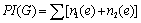Archive
Special IssuesVolume 1, Issue 1, December 2016, Page: 1-4
P. Gayathri, Department of Mathematics, A. V. College (Autonomous), Mayiladuthurai, Tamilnadu, India
K. R. Subramanian, Department of Computer Applications, Shrimati Indira Gandhi College, Trichy, Tamilnadu, India
Received: Sep. 19, 2016;       Accepted: Nov. 18, 2016;       Published: Dec. 26, 2016
Abstract
The Padmakar – Ivan (PI) index of polyominoes is examined.Efficient calculations of formulas for PI index for the polyominoes are put forward. In chemical graph theory, the PI index is a topological indexof a graph G is defined as, where for edge e = xy, n1 (e) is the number of edges of G lying closer to x than y, n2 (e) is the number of edges of G lying closer to y than x and summation goes over all edges of G. The edges equidistant from x and y are not considered for the calculation of PI index. In this paper, we calculated the PI index of polyominoes like square Polyomino, L-Polyomino,T-Polyomino, Straight-Polyomino and Skew-Polyomino.
Keywords
Molecular Graph, Polyominoes, Topological Indices, PI (Padmakar-Ivan)Index
P. Gayathri, K. R. Subramanian, The PI(Padmakar-Ivan) Index of Polyominoes, International Journal of Discrete Mathematics. Vol. 1, No. 1, 2016, pp. 1-4. doi: 10.11648/j.dmath.20160101.11
Reference

D. A. Klarner, Polyominoes, in: J.E. Goodman, J. O’Rourke (Eds.), Handbook of Discrete and Computational Geometry, CRC Press LLC, 1997, pp. 225–242 (Chapter 12).

P.E. John, P.V. Khadikar, J. Singh, A method of computing the PI index of benzenoid hydrocarbons using orthogonal cuts, J. Math. Chem. 42(1) (2007) 37–45.

S. W. Golomb, Polyominoes, Charles Scribner’s Sons, 1965.

S. W. Golomb, Checker boards and polyominoes, Amer. Math. Monthly 61 (1954) 675–682.

H. Wiener, Structural determination of paraffin boiling points, J. Amer. Chem. Soc. 69 (1947) 17–20.

R. Todeschini, V. Consodni, Handbook of Molecular Descriptors, Willey-VCH, Weinheim, 2000.

I. Gutman, A formula for the Wiener number of trees and its extension to graphs containing cycles, Graph Theory Notes New York 27 (1994)9–15.

P. V. Khadikar, N. V. Deshpande, P. P. Kale, A. Dobrynin, I. Gutman, G. J. Domotor, The Szeged index and an analogy with the Wiener index, J. Chem. Inform. Comput. Sci. 35 (1995) 547–550.

P. V. Khadikar, P. P. Kale, N. V. Deshpande, S. Karmarkar, V.K. Agrawal, Novel PI indices of hexagonal chains, J. Math. Chem. 29 (2001)143–150.

P. V. Khadikar, S. Karmarkar, V. K. Agrawal, A novel PI index and its applications to QSRP/QSAR studies, J. Chem. Inf. Comput. Sci. 41 (4)(2001) 934–949.

P. V. Khadikar, P. P. Kale, N. V. Deshpande, S. Karmarkar, V.K. Agrawal, Novel PI indices of hexagonal chains, J. Math. Chem. 29 (2001)143–150.

P. V. Khadikar, A. Phadnis, A. Shrivastava, QSAR study on toxicity to aqueous organism using PI index, Bioorg. Med. Chem. 10 (2002)1181–1188.

H. Deng, Extremal catacondensed hexagonal systems with respect to the PI index, MATCH Commun. Math. Comput. Chem. 55 (2) (2006)453–460.

H. Deng, S. Chen, The PI index of pericondensed benzenoid graphs, J. Math. Chem. (2006), doi:10.1007/s10910-006-9175-9.

H. Deng, The PI index of TUVC6[2p, q], MATCH Commun. Math. Comput. Chem. 55 (2) (2006) 461–476.

H. Deng, S. Chen, J. Zhang, The PI index of Phenylenes, J. Math. Chem. 41 (1) (2007) 63–69.

L. Alonso, R. Cerf, The three dimensional polyominoes of minimal area, Electron. J. Combin. 3 (1996) 39p.

I. Gutman, N. Trinajstic, Graph theory and molecular orbitals, total π-electron energy of alternant hydrocarbons, Chem. Phys. Lett. 17 (1972) 535–538.

I. Gutman, K.C. Das, The first Zagreb index 30 years after, MATCH Commun. Math. Comput. Chem. 50 (2004) 83–92.

S. Nikolic, G. Kovačevic, A. Miličevic, N. Trinajstic, The Zagreb indices 30 years after, Croat. Chem. Acta 76 (2003) 113–124.

B. Zhou, I. Gutman, Relations between Wiener, hyper-Wiener and Zagreb indices, Chem. Phys. Lett. 394 (2004) 93–95.

B. Zhou, Zagreb indices, MATCH Commun. Math. Comput. Chem. 52 (2004) 113–118.

B. Zhou, I. Gutman, Further properties of Zagreb indices, MATCH Commun. Math. Comput. Chem. 54 (2005) 233–239.

D.A. Klarner, Polyominoes, in: J.E. Goodman, J. O’Rourke (Eds.), Handbook of Discrete and Computational Geometry, CRC Press LLC, 1997, pp. 225–242 (Chapter 12).

Y. Zeng, F. Zhang, Extremal polyomino chains on k-matchings and k-independent sets, J. Math. Chem. 42 (2) (2007) 125–140.

L. Xu, S. Chen, The PI index of polyomino chains, Appl. Math. Lett. 21 (2008) 1101–1104.

J. Yang, F. Xia, S. Chen, On the Randic index of of polyomino chains, Appl. Math. Sci. 5 (5) (2011) 255–260.

J. Yang, F. Xia, S. Chen, On sum-connectivity index of polyomino chains, Appl. Math. Sci. 5 (6) (2011) 267–271.